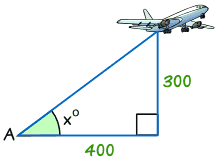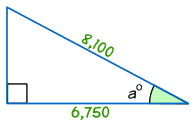# 求直角三角形的角度

## 例子

、看看已知的边是

• 边（就是：我们想求的角的其中一边，但不是最长的边），
• 边（就是：对着我们想求的角的边），或
• 边（就是：最长的边）

### 例子：在这个梯子的例子，我们知道：

• 角 "x" 的 边的长度：2.5
• 最长的边（边）的长度：5

、用以下的公式来决定用正弦、余弦 正切：

 正弦 sin(θ) = 对边 / 斜边 余弦 cos(θ) = 邻边 / 斜边 正切 tan(θ) = 对边 / 邻边

、把已知值代入正弦方程：

Sin (x) = 边 / 边 = 2.5 / 5 = 0.5

、解方程！

sin(x) = 0.5

x = sin-1(0.5)

x = 30°

### 但……sin-1 的意思是什么？sin-1（叫 "反正弦"）是正弦的相反……
……它以 "对边/斜边" 的为输入来计算角度。

### 例子：

• 正弦函数：sin(30°) = 0.5
• 反正弦函数：sin-1(0.5) = 30°在计算器上，按以下的键（视乎计算器的牌子）： '2ndF sin' 或 'shift sin'。

## 逐步来

• 、看看已知的边是对边、邻边还是斜边。
• 、用上面的公式来决定用 正弦、余弦正切。
• 、正弦：求 对边/斜边，余弦：求 邻边/斜边,正切：求 对边/邻边。
• 、用计算器来求角度，用 sin-1、cos-1tan-1

## 例子### 例子

• 、已知的边是 边 (300) 和 边 (400)。
• 、从上面的公式，我们知道应该用 切。
• 、计算 对边/邻边 = 300/400 = 0.75
• 、用计算器的 tan-1 键来求角度

Tan x° = 对边/邻边 = 300/400 = 0.75

tan-1 of 0.75 = 36.9° （保留一位小数）### 例子

• 、已知的边是 变 (6,750) 和 边 (8,100)。
• 、从上面的公式，我们知道应该用 弦。
• 、计算 邻边/斜边 = 6,750/8,100 = 0.8333
• 四、用计算器来算 cos-1(0.8333) ：

cos a° = 6,750/8,100 = 0.8333

cos-1(0.8333) = 33.6° (保留一位小数）